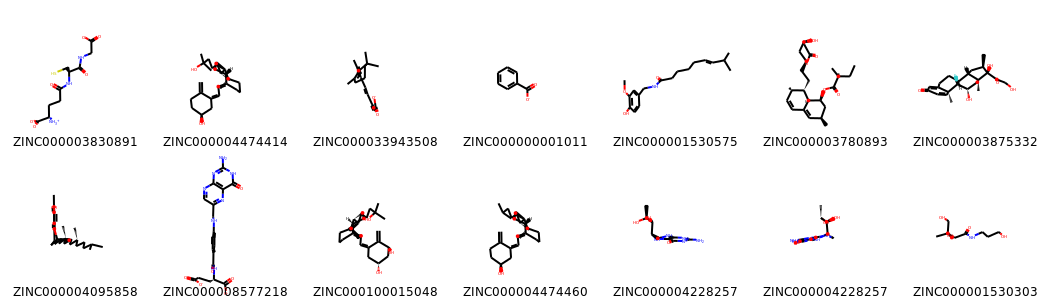# Building a Multi-Molecule Mol2 reader for RDKit V2¶

In this mini-tools entry, I want to introduce a simple but power modification to my previous version of Mol2MolSupplier for RDKIT:

Perhaps some of you (as me) encountered errors when using the previous version of the Mol2MolSupplier.

Well, this modification overcomes many of the previous errors which were differences in the headers and order of the Mol2 molecule blocks in different Mol2 files.

This new version used the same approach as before but in a simpler and faster way.

Let's go directly to the new function:

## Importing the libraries¶

In :
from rdkit import Chem
from rdkit.Chem import Draw,AllChem
from rdkit.Chem.Draw import IPythonConsole

import pandas as pd

In :
def Mol2MolSupplier (file=None,sanitize=True):
mols=[]
with open(file, 'r') as f:

start=[index for (index,p) in enumerate(doc) if '@<TRIPOS>MOLECULE' in p]
finish=[index-1 for (index,p) in enumerate(doc) if '@<TRIPOS>MOLECULE' in p]
finish.append(len(doc))

interval=list(zip(start,finish[1:]))
for i in interval:
block = ",".join(doc[i:i]).replace(',','')
m=Chem.MolFromMol2Block(block,sanitize=sanitize)
mols.append(m)
return(mols)


I will use the following multi-molecule mol2 file to show how the function works. The file contains 169 different molecules from ZINC.

In :
filePath ='for-sale+in-man+fda+named+endogenous.mol2'

In :
database=Mol2MolSupplier(filePath,sanitize=True)

RDKit WARNING: [12:48:54] ZINC000003801919: warning - O.co2 with non C.2 or S.o2 neighbor.


Because we are using RDKit to convert mol2 block texts to RDKit molecules, we can use sanitization or not. Moreover, we can see the warning for sanitization problems. If a molecule is not valid we will get a 'None' element.

In :
database[:10] #The first 10 elements in the list

Out:
[None,
None,
None,
None,
None,
None,
None,
None,
<rdkit.Chem.rdchem.Mol at 0x7f4d27fc4c10>,
<rdkit.Chem.rdchem.Mol at 0x7f4d27e57df0>]

Once molecules loaded. We can perform any calculation available in RDKit or converting the molecules to other formats (e.g. SDF). For instance, creating a pandas table with some useful molecular information.

In :
table=pd.DataFrame()
index=0
for mol in database:
if mol:
table.loc[index,'Name']=mol.GetProp('_Name')
table.loc[index,'NumAtoms']=mol.GetNumAtoms()
table.loc[index,'SMILES']=Chem.MolToSmiles(mol)
index=index+1

In :
table.head(10) #The first 10 non None elements in the list

Out:
Name NumAtoms SMILES
0 ZINC000003830891 20.0 [NH3+][C@@H](CCC(=O)N[C@@H](CS)C(=O)NCC(=O)[O-...
1 ZINC000004474414 29.0 C=C1CC[C@H](O)C/C1=C/C=C1\CCC[C@@]2(C)[C@H]1CC...
2 ZINC000033943508 22.0 CC1=C(/C=C/C(C)=C\C=C\C(C)=C/C(=O)[O-])C(C)(C)...
3 ZINC000000001011 9.0 O=C([O-])c1ccccc1
4 ZINC000001530575 22.0 COc1cc(CNC(=O)CCCC/C=C/C(C)C)ccc1O
5 ZINC000003780893 30.0 CCC(C)(C)C(=O)O[C@H]1C[C@@H](C)C=C2C=C[C@H](C)...
6 ZINC000003875332 28.0 C[C@@H]1C[C@H]2[C@@H]3CCC4=CC(=O)C=C[C@]4(C)[C...
7 ZINC000004095858 31.0 Cc1c(C)c2c(c(C)c1O)CC[C@@](C)(CCC[C@H](C)CCC[C...
8 ZINC000008577218 32.0 Nc1nc2ncc(CNc3ccc(C(=O)N[C@@H](CCC(=O)[O-])C(=...
9 ZINC000100015048 30.0 C=C1/C(=C\C=C2/CCC[C@@]3(C)[C@H]2CC[C@@H]3[C@H...

Drawing some non None molecules keeping the 3D coordinates from the mol2 file.

In :
no_none=[mol for mol in database if mol] # None element can´t be drawn, this loop keep only valid entries
[Chem.SanitizeMol(mol) for mol in no_none]
Draw.MolsToGridImage(no_none[:14],molsPerRow=7,subImgSize=(150,150),legends=[mol.GetProp('_Name') for mol in no_none[:14]],maxMols=100)

Out:In :
# Drawing 3 random molecules of non None list
Draw.IPythonConsole.drawMol3D(no_none)
Draw.IPythonConsole.drawMol3D(no_none)
Draw.IPythonConsole.drawMol3D(no_none)


You appear to be running in JupyterLab (or JavaScript failed to load for some other reason). You need to install the 3dmol extension:
jupyter labextension install jupyterlab_3dmol

You appear to be running in JupyterLab (or JavaScript failed to load for some other reason). You need to install the 3dmol extension:
jupyter labextension install jupyterlab_3dmol

You appear to be running in JupyterLab (or JavaScript failed to load for some other reason). You need to install the 3dmol extension:
jupyter labextension install jupyterlab_3dmol